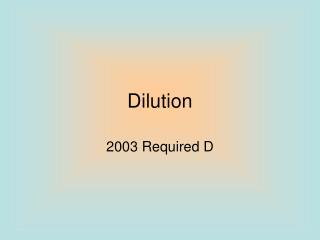DownloadDownload PresentationDilution

# Dilution

Download Presentation## Dilution

- - - - - - - - - - - - - - - - - - - - - - - - - - - E N D - - - - - - - - - - - - - - - - - - - - - - - - - - -
##### Presentation Transcript

1. Dilution 2003 Required D

2. Information Given • A student is instructed to determine the concentration of a solution of CoCl2 based on absorption of light (spectrometric/ colormetric method). The student is provided with a .1 M solution of CoCl2 with which to prepare standard solutions with concentration of .02 M, .04 M, .06 M and .08 M.

3. Questions…. • Part A: • Describe the procedure for diluting the .1 M solution to a concentration of .02 M using distilled water, a 100 mL volumetric flask, and a pipet or buret. Include specific amounts where appropriate.

4. Dilution: How To • To dilute: • You are changing the concentration but keeping the same substance of a solution. Therefore, you should just focus on the units and concentration to work out the problem as well as moles. • Remember!!!! M stands for concentration of solution, M1 is the initial while M2 is how much you want. V1 is the original volume while V2 is the new amount needed, remembered this is the total new amount not the amount added

5. Dilution Then you would solve for x. This would be the amount of liters that you need of the .1 M solution. Then once gaining this amount of .02 liters or 20 mL, this is the amount of .1 M of CoCl2 you need to first put into the volumetric flask. After you fill this up with 80 mL of water it would be the total to fill up the volumetric flask.

6. Questions….. • Part B: • Identify the optimum wavelength for the analysis. • Optimal wavelength is the maximum absorbance at a wavelength (self explanatory). Looking at the graph, it is clear to see that the maximum absorbance is at about 510 nm.

7. Questions…. • Part C: • The absorbance of the unknown solution is .275. What is the concentration of the solution? • Use the graph to determine the concentration. The concentration would be .05 M.

8. Questions…. • Part D: • Beer’s Law is an expression that includes three factors that determines the amount of light that passes through a solution. Identify two of these factors. • What is Beer’s Law? • determines the quantitatively analysis using spectroscopy. Spectroscopy studies em radiation emitted or absorbed by chemical species.

9. Beer-Lambert Law Is molar absorptivity or molar extinction coefficient (L/mol*cm) Length of path, distance light travels through the solution Concentration of solution (M)

10. Questions… • Therefore three of the factors are just the components of the equation for Beer’s Law. • molar absorptivity or molar extinction coefficient (L/mol*cm) • Path length, distance light travels through solution • Concentration of absorbing species

11. Questions…. • Part E: • The student handles the sample container that holds the unknown solution and leaves fingerprints in the path of the light beam. How will this affect the calculated concentration of the unknown? Explain your answer. • The fingerprints will not allow the light to go through and distort it somewhat. Therefore the light would be scattered making the detector get less light. This would increase the absorbance making an increased concentration from Beer’s Law.

12. Questions… • Absorbance • Is defined as base 10 log of the reciprocal of the transmittance of a pure solvent. I-intensity of light at specified wavelength I0- intensity of light before it enters the sample I/I0- -transmittance

13. Questions…. • Part F: • Why is this method of determining the concentration of CoCl2 whereas using the same method for measuring the concentration of NaCl would not be appropriate? • Na+ doesn’t absorb energy in the visible spectrum, but Co2+ does its pink colored. NaCl-clear CoCl2 -pink

14. Wrap Up • For this problem: • Dilution • Beer’s Law • Reading Graphs • Some solution color information